# HC Verma Solutions Class 12 Chapter 19 Electric Current Through Gases

HC Verma Solutions Class 12 Chapter 19 Electric Current Through Gases have been provided here mainly to help students boost their scoring potential and at the same be proficient in solving problems given in the chapter. The solutions consist of detailed and well-written answers that will enable students to learn as well as understand topics more clearly. Students can, therefore, prepare in a much effective manner especially for exams like JEE. Meanwhile, students will get to learn topics of electric current in problems such as:

• Finding the distance travelled by the particle if its energy, force, and acceleration, velocity are given and vice versa.
• Finding the pressure of a tube and questions related to cathode acting as a black body.
• Questions on amplification factor, load resistance, mutual conductance and much more.

The HC Verma Solutions covers all the exercises given in this chapter. If students are stuck or they are unable to get the right result, then they can make use of the solutions to learn the right method as well as find the accurate answer. This will also help them to study more productively and perform well in the exams.

## Maor Topics Related To Electric Current Through Gases

In this chapter, students will come across topics such as;

• Discharge through Gasses at Low Pressure
• Cathode Rays
• Canal Rays or Positive Rays
• Discovery and Properties of Electron
• Thermionic Emission
• Diode Valve
• Triode Valve

## Class 12 Important Questions In Chapter 19

1. Suppose we connect an AC source to a diode and a resistor in series. What nature of current will flow through the resistor? Will it be AC or DC?

2. When we heat an isolated metal sphere at high temperature will it become positively charged due to thermionic emission? State your observations.

3. Thermionic emission does not take place in nonconductors. Explain.

4. When will the plate current in a triode valve be maximum? When the potential of the grid is;

(a) nonpositive (b) negative (c) zero (d) positive

5. Can you state which is true for cathode ray?

(a) Travels along straight lines (b) X-ray is emitted when it strikes a metal surface (c) Is a type of electromagnetic wave (d) No effect of the magnetic field

## HC Verma Solutions Vol 2 Electric Current Through Gases Chapter 19

Question 1: A discharge tube contains helium at a low pressure. A large potential difference is applied across the tube. Consider a helium atom that has just been ionized due to the detachment of an atomic electron. Find the ratio of the distance travelled by the free electron to that by the positive ion in a short time dt after the ionization.

Solution:

Let the mass of the electron and positive helium ion be me and mh.

me = 9.1×10-31kg and mh = 4×mass of proton = 4×1.6×10-27kg

Magnitude of force experienced by electron: F = qE

=> me a = qE

=> a = qE/me

and Magnitude of force experienced by positive helium ion: F = qE

=> mh a = qE

=> a = qE/mh

We know, s = ut + at2/2

Since initial velocity of electron and helium ion is zero.

=> s = at2/2 …(1)

Distance travelled by free electron and positive helium ion for dt duration:

(using (1))

se = qE/2me (dt)2 and

sh = qE/2mh (dt)

The required ratio is: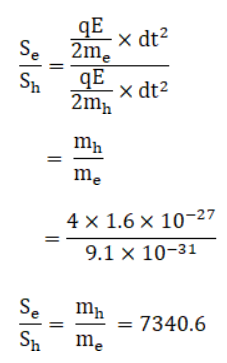Question 2: A molecule of a gas, filled in a discharge tube, gets ionized when an electron is detached from it. An electric field of 5.0 kV m–1 exists in the vicinity of the event.

(a) Find the distance travelled by the free electron in 1 μs assuming no collision.

(b) If the mean free path of the electron is 1.0 mm, estimate the time of transit of the free electron between successive collisions

Solution:

(a) Electric field =E = 5 kV m-1 = 5×103 V m-1

Time = t = 1 μs = 1×10-6 s.

Let m be the mass of electron and q be the charge of electron.

where m = 9.1×10-31 kg and q = 1.6×10-19 C

We know, F = qE and F= ma

on comparing, we get

=> ma = qE

=> a = qE/m

Also, distance travelled by an electron, at u = 0 m/s

=> s = (1/2) at2

=> s = (1/2) (qE/m) t2

=> s = [1.6×10-19x5x103x10-12]/[2×9.1×10-31]

=> s = 439.5 m

(b) Mean free path S travelled by electron, s = 1mm = 10-3 m

a = qE/m = [1.6×10-19x5x103]/[9.1×10-31] = 0.87×1015

Also, s = (1/2) at2

Or t2 = 2s/a = [2 x 10-3]/[ 0.87×1015] = 2.29×10-18

Or t = 1.51 x 10-9s = 1.5 ns

Question 3: The mean free path of electrons in the gas in a discharge tube is inversely proportional to the pressure inside it. The Crookes dark space occupies half the length of the discharge tube when the pressure is 0.02 mm of mercury. Estimate the pressure at which the dark space will fill the whole tube.

Solution:

Let the mean free path be L and pressure be P.

When P becomes half, L doubles, i.e. the whole tube is filled with Crook’s dark space.

Thus, the required pressure = 0.02/2 = 0.01 m of Hg.

Question 4: Two discharge tubes have identical material structure and the same gas is filled in them. The length of one tube is 10 cm and that of the other tube is 20 cm. Sparking starts in both the tubes when the potential difference between the cathode and the anode is 100 V. If the pressure in the shorter tube is 1.0 mm of mercury, what is the pressure in the longer tube?

Solution:

Let d1 and p1 be the length and pressure of short tube.

And, d2 and p2 be the length and pressure of long tube.

So, p1 = 1.0 mm , d1 = 10 cm and d2 = 20 cm

According to Paschen’s law, V = f pd

Sparking potential of a gas in a discharge tube is the function of the product of pressure of the gas and separation between the electrodes.

V = p1d1 = p2d2

=> p2 = p1d1/d2 = [1×10]/20 = 0.5 mm

Question 5: Calculate n(T)/n (1000 K) for tungsten emitter at T = 300 K, 2000 K and 3000 K where n(T) represents the number of thermions emitted per second by the surface at temperature T. Work function of tungsten is 4.52 eV.

Solution:

According to Richardson-Dushman Equation thermionic current:

i = ne = AST2 e-φ/kT

where, A is the constant depend on nature of metal, S is the surface area, n is the thermions emitted, k is the Boltzmann constant, T is the absolute temperature and φ is the work function.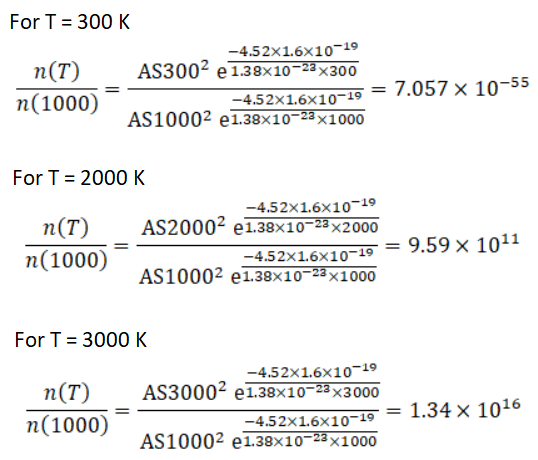Question 6: The saturation current from a thoriated-tungsten cathode at 2000 K is 100 mA. What will be the saturation current for a pure-tungsten cathode of the same surface area operating at the same temperature? The constant A in the Richardson-Dushman equation is 60 × 104 A m-2K-2 pure tungsten and 3.0 × 104 A m-2K-2 for thoriated tungsten. The work function of pure tungsten is 4.5 eV and that of thoriated tungsten is 2.6 eV.

Solution:

According to Richardson-Dushman Equation thermionic current:

i = ne = AST2 e-φ/kT

where, A is the constant depend on nature of metal, S is the surface area, n is the thermions emitted, k is the Boltzmann constant, T is the absolute temperature and φ is the work function.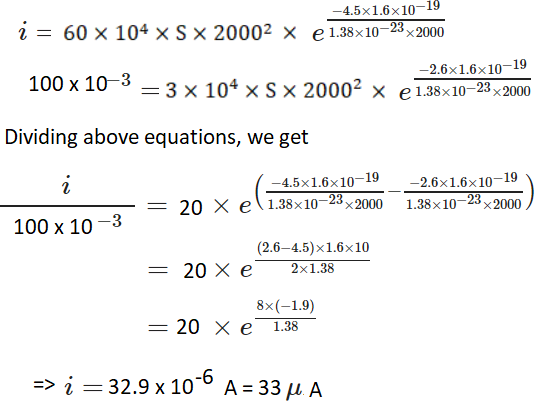Question 7: A tungsten cathode and a thoriated-tungsten cathode have the same geometrical dimensions and are operated at the same temperature. The thoriated-tungsten cathode gives 5000 times more current than the other one. Find the operating temperature. Take relevant data from the previous problem.

Solution:

According to Richardson-Dushman Equation thermionic current:

i = ne = AST2 e-φ/kT

where, A is the constant depend on nature of metal, S is the surface area, n is the thermions emitted, k is the Boltzmann constant, T is the absolute temperature and φ is the work function.

=> Ittungsten = 5000 Iptungsten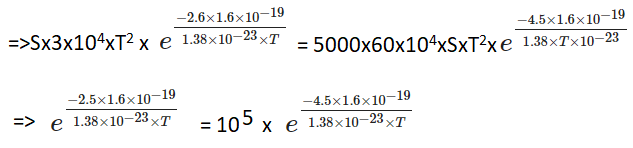Applying “ln” both sides to eliminate exponential function.

[-2.89×104]/T = 11.51 + [-5.22 x104]/T

=> T = 2024 K

Question 8: If the temperature of a tungsten filament is raised from 2000 K to 2010 K, by what factor does the emission current change? Work function of tungsten is 4.5 eV.

Solution:

Let initial and final temperature of tungsten filament be I1 and I2, then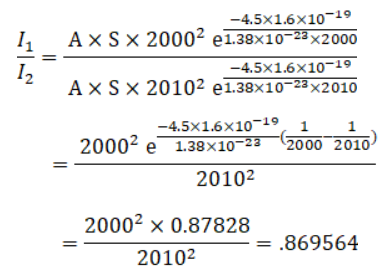The required factor is I2/I1 = 1/0.8696 = 1.14 (approx)

Question 9: The constant A in the Richardson-Dushman equation of tungsten is 60 × 104 A m-2 K-2. The work function surface area 2.0 × 10–5 m2 is heated by a 24 W electric equals the energy input by the heater and the temperature becomes constant. Assuming that the cathode radiates like a blackbody, calculate the saturation current due to thermions. Take Stefan constant =6 × 10-8 W m-2 K-4. Assume that the thermions take only a small fraction of the heat supplied.

Solution:

The power radiated: P = SσT4

[Using Stefen’s law]

=> 24 = 6 x 10-8 x 2 x 10-5 x T4

=> T = 2.1147 x 103 K

Again, According to Richardson-Dushman Equation thermionic current i

i = ne = AST2 e-φ/kT

=>i = 60 x 104 x 2×10-5 x 2114.72 x e^{ [-4.5×1.6×10-19]/[1.38 x10-23x2114.7]}

=> i = 1.034 x 10-3 A = 1 mA (approx.)

Question 10: A plate current of 10 mA is obtained when 60 volts are applied across a diode tube. Assuming the Langmuir-Child relation ip ∝ VP(3/2) to hold, find the dynamic resistance rp in this operating condition.

Solution:

Given: ip ∝ VP(3/2)

If k = proportionality constant , then

ip = k VP(3/2) ……………..(1)

Derivate above equation with respect to ip, we have

1 = (3/2) k(VP)(1/2) x dVP/dIP …(2)

Dividing (2) by (1)

1/iP = (3/2V) dVP/dIP

At Vg = constant

=> (dVP/dIP) = 2V/3iP = [2×60]/[3x10x10-3] = 4 k ohms

Question 11: The plate current in a diode is 20 mA when the plate voltage is 50 V or 60 V. What will be the current if the plate voltage is 70V?

Solution:

For a given temperature current remains same for all voltages i.e. for 70 V current is 20 mA.

Question 12: The power delivered in the plate circuit of a diode is 1.0 W when the plate voltage is 36 V. Find the power delivered if the plate voltage is increased to 49 V. Assume Langmuir – Child equation to hold.

Solution:

Let ip1 is the plate current and Vp1 is the plate voltage.

Here P1 = 1.0 W, Vp1 = 36 V, Vp2 = 49 V

We know, P = VI

=> P1 = V1 ip1

=> ip1 = P1/V1 = 1/36

Using Langmuir-Child equation, we have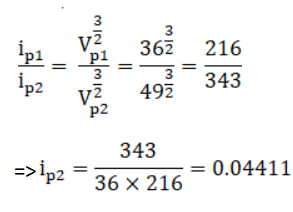P2 = iP2 x VP2 = 0.04411 × 49 = 2.16

Or P2 = 2.2 W

Question 13: A triode valve operates at Vp = 225 V and Vg = –0.5 V. The plate current remains unchanged if the plate voltage is increased to 250 V and the grid voltage is decreased to –2.5 V. Calculate the amplification factor.

Solution:

We know, amplification factor for triode = – (ΔVp/ΔVg)

Here ΔVp = ΔVp1 – ΔVp2 = 225 – 250 = -25

ΔVg = ΔVg1 – ΔVg2 = -0.5 + 2.5 = 2

=> amplification factor for triode = 12.5

Question 14: Calculate the amplification factor of a triode valve which has plate resistance of 2 kΩ and transconductance of 2 millimho.

Solution:

rp = 2 KΩ = 2× 103 (plate resistance)

gm =2 millimho = 2× 10-3 mho(transconductance)

Amplification factor for triode = μ = rp x gm = 2 x 103 x 2 x 10-3 = 4

Question 15: The dynamic plate resistance of a triode valve is 10 kΩ. Find the change in the plate current if the plate voltage is changed from 200 V to 220 V.

Solution:

The dynamic resistance of triode

rp = (ΔVp/ΔIp) [at Vg = constant)

=>104 = [220-200]/ ΔIp

=> ΔIp = 20/104 = 2 mA

Question 16: Find the values of rp, μ and gm of a triode operating at plate voltage 200 V and grid voltage –6 V. The plate characteristics are shown in figure.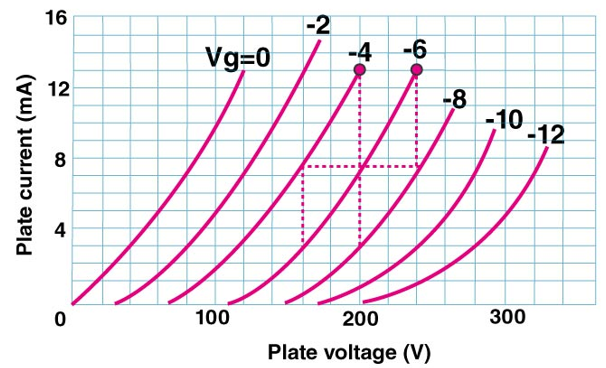Solution:

The dynamic resistance of triode

rp = (ΔVp/ΔIp) [at Vg = constant)

From figure, considering the line Vg = -6 V, we get two value of VP i.e.

VP1 = 160 V and VP2 = 240 V

ip1 = 3 mA and ip2 = 13 mA

=> rp = [240-160]/[(13-3)x10-3] = 8 K ohm

Mutual inductance gm of a triode valve:

gm = (ΔIp/ΔVg) [at Vp = constant)

Taking any two points on the 200 V line, in the graph,

Vp = 200 V, ip1 = 13 mA, ip2 = 3mA and Vg1 = -4 V, Vg2 = -8 V

=>gm = [(13-3)x10-3]/(-8+4) = 2.5 milli mho

Amplification factor for triode:

μ = (ΔVp/ΔVG) [at Ip = constant)

μ = (100-180)/(-6-(-10) = 20

Question 17: The plate resistance of a triode is 8 kΩ and the transconductance is 2.5 milli mho.

(a) If the plate voltage in increased by 48 V, and the grid voltage is kept constant, what will be the increase in the plate current?

(b) With plate voltage kept constant at this increased value, how much should the grid voltage be decreased in order to bring the plate current back to its initial value?

Solution:

(a) The dynamic resistance of triode:

rp = (ΔVp/ΔIp) [at Vg = constant)

When ΔVP = 48 V, Vg = Constant

=>8000 = 48/ΔIp

= ΔIp = 6 mA

(b) When gm = 2.5 millimho = 0.0025 mho and

ΔIp = 6 mA = 0.006 A

gm = (ΔIp/ΔVg) [at Vp = constant)

=>0.0025 = 0.006/ΔVg

=> ΔVg = 2.4 V

Question 18: The plate resistance and the amplification factor of a triode are 10 kΩ and 20. The tube is operated at plate voltage 250 V and grid voltage –7.5 V. The plate current is 10 mA

(a) To what value should the grid voltage be changed so as to increase the plate current to 15 mA?

(b) To what value should the plate voltage be changed to take the plate current back to 10 mA?

Solution:

(a) Here ip2 = 15 mA ; VP = Constant, and ip1 = 10 mA

Find: Vg2 = ?

We know, gm = μ/rp

=>gm = 20/10000 = 2 x 10-3 mho

Also,

gm = (ΔIp/ΔVg) [at Vp = constant)

0.002 = [(15-10)x10-3]/ΔVg

=> ΔVg = 0.005/0.002 = 2.5 V

Also we have ΔVg = Vg2 – Vg1

=> Vg2 = 2.5 – 7.5 = -5 V

(b) The dynamic resistance of triode:

rp = (ΔVp/ΔIp) [at Vg = constant)

Here ip3 = 10 mA, ip2 = 15mA and ip3 = 10mA

Find : VP3

=>10000 = ΔVp/[(10-15)x10-3]

= ΔVp = -50

Also, ΔVp = Vp3 – Vp2

(Given : VP2 = 250 V)

=> Vp3 = 200 V

Question 19: The plate current, plate voltage and grid voltage of a 6F6 triode tube are related as

ip = 41(Vp + 7 Vg)1.41, Where Vp and Vg are in volts and ip in microamperes. The tube is operated at Vp = 250 V, Vg = –20 V. Calculate

(a) the tube current,

(b) the plate resistance,

(c) the mutual conductance and

(d) the amplification factor.

Solution:

(a) Here, VP = 250 V, Vg = –20 V

And, ip = 41(Vp + 7 Vg)1.41 …..(1)

=> ip = 41(250 – 140)1.41 = 30984.71 μA = 30 mA

b) Differentiating equation (1) w.r.t. ip

1 = 41 x 1.41(Vp + 7 Vg) 1.41 x dVp/dip ….(2)

[Here, Vg = constant]

The dynamic resistance of triode, rp = dVp/dip

When , Vg = constant

From equation (2),

dVp/dip = [1×106]/[41×1.41×1100.41] = 2.5 K Ω

(c) Differentiating equation (1) w.r.t. Vg

dip/dVg = 41 x 1.41(Vp + 7 Vg)1.41 x 7

where, Vp constant

We know, gm = dip/dVg at Vp constant

=> 41 x 1.41 x 1100.41 x 7 = 2780.18 μ mho = 2.78 millimho

(d) Amplification factor for triode = μ = rp x gm

= 2.5 x 103 x 2.78 x 10-3

= 6.95

μ = 7 (approx.)

Question 20: The plate current in a triode can be written as ip = k(Vg + Vp/μ )3/2. Show that the mutual conductance is proportional to the cube root of the plate current.

Solution:

Given: ip = k(Vg + Vp/μ )3/2

We know, gm = dip/dVg at Vp constant

Where, gm is the mutual conductance, ip is the plate current, Vp is the plate current and Vg is the grid voltage.

gm = dip/dVg = (3/2)k[Vg + Vp/μ](1/2)

Cubing both the sides, we get

gm3 = (27/8)k3[Vg + Vp/μ](3/2) = (27/8) k2ip

=>gm ∝ ip1/3

Hence proved.

Question 21: A triode has mutual conductance = 2.0 millimho and plate resistance = 20 kΩ. It is desired to amplify a signal by a factor of 30. What load resistance should be added in the circuit?

Solution:

A triode has mutual conductance = 2.0 millimho and plate resistance = 20 kΩ.

gm = 2.0 millimho = 2×10-3 mho and rp = 20Ω and A = 30

We know, μ = rp x gm = 20 x 103 x 2 x 10-3 = 40

and rP/RL = 20000/RL

Also, we know

A (Voltage gain) = μ/[1+ rP/RL]

Substituting the values, we have

30 = 40/[1+20000/RL]

=> RL = 60 kΩ

Question 22: The gain factor of an amplifier is increased from 10 to 12 as the load resistance is changed from 4 kΩ to 8 KΩ. Calculate (a) the amplification factor and (b) the plate resistance.

Solution:

We know,

A (Voltage gain) = μ/[1+ rP/RL] ….(1)

When:

A1 = 10, Rl1 = 4kΩ

(1)=> 10 = μ/[1+rp/4000]

=>10(4000 + rp) = 4000 μ

Or 400 μ – rp = 4000 …(2)

When: A2 = 12, Rl2 = 8kΩ

(1)=> 12 = μ/[1+rp/8000]

=>12(8000 + rp) = 8000 μ

Or 8000 μ – 12rp = 96000 …(3)

Solving, (2) and (3), we have

μ = 15 and rp is 2000Ω or 2 kΩ.

Question 23: Figure shows two identical triode tubes connected in parallel. The anodes are connected together, the grids are connected together and the cathodes are connected together. Show that the equivalent plate resistance is half to the individual plate resistance. The equivalent mutual conductance is double the individual mutual conductance and the equivalent amplification factor is the same as the individual amplification factor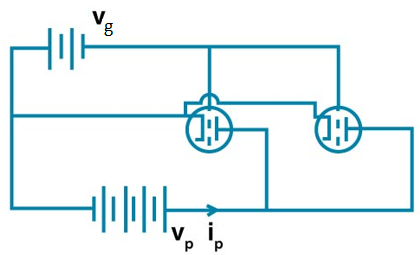Solution:

Given the anodes are connected together, and two triodes have same voltage and same current, this implies, rP1 = rP2 = r

The equivalent resistance,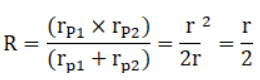Let gm1 and gm2 be the individual conductance.

From figure, gm1 = gm2 = g.

Since, two triodes are parallel, we have equivalent conductance.

=> G = gm1 + gm2 = g + g = 2g

Now, μ1 = gm1 rp1 = gr and μ2 = gm2 rp2 = gr

So, equivalence amplification factor = μ = RG = (r/2) x 2g = rg

Therefore, equivalent amplification factor is the same as the individual amplification factor.